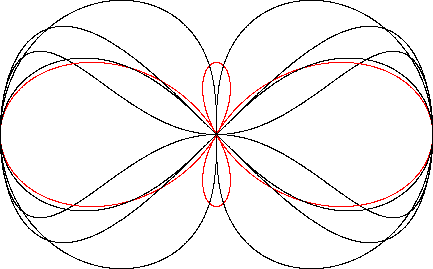Bowtie
BOWTIE

Balmoral Software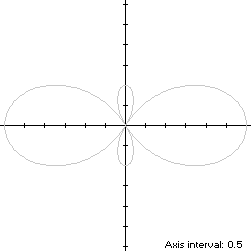The bowtie is a figure-8 curve with polar equation
p(t) = 2 sin(2t) + 1, 0 ≤ t < 2π
Rotating the curve clockwise by π/4 produces the equivalent bisymmetric curve S with polar equation
r(t) = p(t + π/4) = 2 cos(2t) + 1, 0 ≤ t < 2π
The corresponding implicit function can be found by replacing cos(t) with x/r and r with x2 + y2:
(x2 + y2)3 = (3x2 - y2)2
The corresponding parametric functions are:
x(t) = 2cos(t) + cos(3t)

y(t) = sin(3t)

Movement along S is always counterclockwise, crossing the origin four times. At t = 0, the path starts at the maximum abscissa point (3,0) and moves through Quadrant I along its right lobe to its maximum ordinate point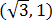at t = π/6, and then on to the origin at t = π/3. It continues along the lower small lobe, returning to the origin at t = 2π/3. Next, it travels along the left lobe through Quadrant II to another maximum ordinate point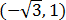at t = 5π/6, then on to its minimum abscissa point (-3,0) at t = π and back to the origin at t = 4π/3. The path continues through the upper small lobe, including a third maximum ordinate point (0,1) at t = 3π/2, the origin and Quadrant IV back to its starting point at t = 2π:
 t: 0 π/6 π/3 π/2 2π/3 5π/6 π 7π/6 4π/3 3π/2 5π/3 11π/6 2π x(t): 3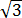0 0 0 --3 -0 0 03 y(t): 0 1 0 -1 0 1 0 -1 0 1 0 -1 0
Since S has three maximum-ordinate points, it is non-convex by the multiple local extrema test. The width x height of the bowtie's bounding rectangle is 6 x 2.

### Metrics

We have
r'(t) = -4 sin(2t),
so by (L2), the perimeter of S is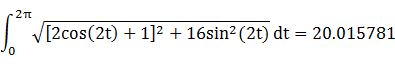and by (A2), the area of S is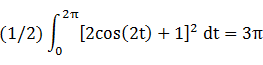### Convex Hull

The convex hull is created by connecting the extreme ordinate points with two horizontal line segments of length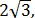each of which is bisected by another extreme ordinate point on one of the small lobes. By (L2), the perimeter of the convex hull is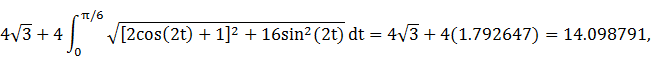which is about 30% shorter than that of the bowtie.

The line segments of the convex hull create two isosceles triangles with the origin, each having an area of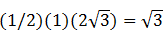as shown in blue in the left diagram below. By (A2), the area of the convex hull is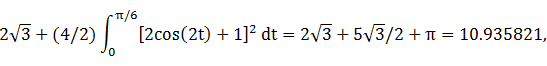which is about 16% more than the area of the bowtie.

### Circumellipse

From Lemma B, we have
x(t)y(t) = [2cos(t) + cos(3t)]sin(3t)
This expression is maximized in the first quadrant at the complicated value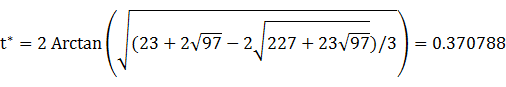The circumellipse dimensions are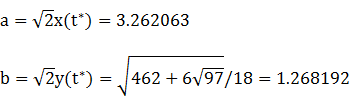For verification, we have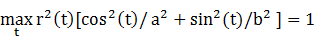### Incircle (lobe)

Consider the right lobe of the bowtie, where -π/3 ≤ t < π/3. The maximum radius of a circle centered on the x-axis and inscribed in the lobe is the maximum ordinate 1 of S, so a candidate for the lobe incircle has radius R = 1 and center abscissa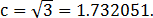The circle must be contained within the lobe, so we require that c - R and c + R both be within the abscissa range [0,3] of the lobe, which is true. For verification, we have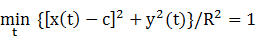### Inellipse (lobe)

Using z = 3 in Lemma E,
d/dt [x(t) - z]y(t) = 2 cos(2t) - 9 cos(3t) + 4 cos(4t) + 3 cos(6t)
has a zero at t* = 0.711832. The corresponding coordinates are
x* = 0.979171

y* = 0.844749

We then have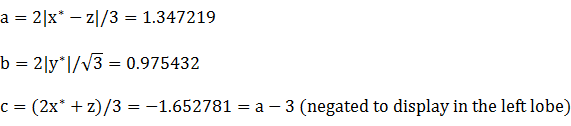For verification, we have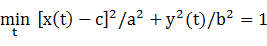### Summary Table

 Perimeter Area Centroid Figure Parameters Incircle (lobe) R = 1 6.283185 3.141593 (1.732051,0) Inellipse (lobe) a = 1.347219b = 0.975432 7.343639 4.128431 (-1.652781,0) Bowtie Width: 6Height: 2 20.015781 9.424778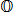Convex hull 14.098791 10.935821 Circumellipse a = 3.262063b = 1.268192 14.930224 12.996524 Circumcircle R = 3 18.849556 28.274334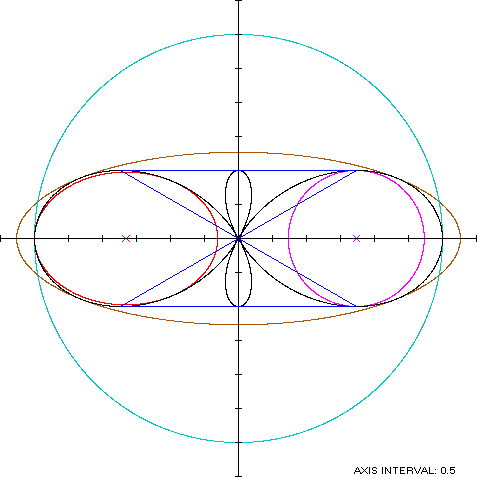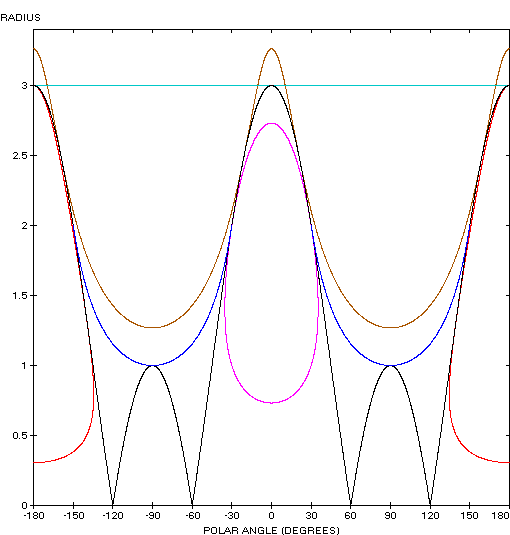The bowtie curve (red) is a member of a group of figure-8 curves described on these pages, including (inside to outside) the dumbbell curve, the Lemniscate of Bernoulli, the Lemniscate of Gerono and the dipole: